##### Actions

(diff) ← Older revision | Latest revision (diff) | Newer revision → (diff)

The orthonormal systemon. It was introduced by H. Rademacher . The functions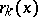are defined by the equations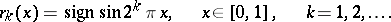Another definition of the Rademacher functionis obtained by considering the binary expansions of numbers in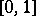: If there is a 0 in the-th place of the binary expansion of, then put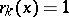, if there is a 1, then put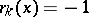, ifor ifadmits two expansions, then put. According to this definition the intervalis partitioned into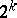equal subintervals, in each of which the functiontakes alternately the values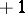and, and at the end points of the subintervals.

The systemis a typical example of a system of stochastically-independent functions and has applications both in probability theory and in the theory of orthogonal series.

One of the major properties of the Rademacher series is shown by Rademacher's theorem: If, then the series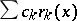converges almost-everywhere on; and the Khinchin–Kolmogorov theorem: If, then the seriesdiverges almost-everywhere on.

Since the Rademacher functions take only the values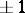on the dyadic irrational points of, the consideration of the seriesmeans that a distribution of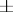signs is chosen for the terms of the series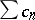, depending on. If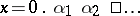is the representation of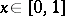as an infinite dyadic fraction, then fora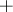sign is placed in front of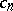and for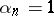asign.

In probabilistic terminology the theorem above means that if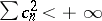, thenconverges for almost-all distributions of signs (converges with probability 1), and if, then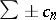diverges for almost-all distributions of signs (diverges with probability 1).

Conversely, a number of theorems in probability theory can be formulated in terms of Rademacher functions. For example, Cantelli's theorem (that for the game of "heads and tails" with stake 1, the average gain tends to zero with probability 1) means that almost-everywhere onthe equalityis satisfied.

How to Cite This Entry: